# 10.4 Polar coordinates: graphs  (Page 6/16)

 Page 6 / 16

## Sketching the graph of a rose curve ( n Even)

Sketch the graph of $\text{\hspace{0.17em}}r=2\mathrm{cos}\text{\hspace{0.17em}}4\theta .$

Testing for symmetry, we find again that the symmetry tests do not tell the whole story. The graph is not only symmetric with respect to the polar axis, but also with respect to the line $\text{\hspace{0.17em}}\theta =\frac{\pi }{2}\text{\hspace{0.17em}}$ and the pole.

Now we will find the zeros. First make the substitution $\text{\hspace{0.17em}}u=4\theta .$

$\begin{array}{c}\text{\hspace{0.17em}}\text{\hspace{0.17em}}\text{\hspace{0.17em}}\text{\hspace{0.17em}}\text{\hspace{0.17em}}\text{\hspace{0.17em}}\text{\hspace{0.17em}}\text{\hspace{0.17em}}\text{\hspace{0.17em}}\text{\hspace{0.17em}}\text{\hspace{0.17em}}\text{\hspace{0.17em}}\text{\hspace{0.17em}}\text{\hspace{0.17em}}\text{\hspace{0.17em}}\text{\hspace{0.17em}}\text{\hspace{0.17em}}\text{\hspace{0.17em}}\text{\hspace{0.17em}}\text{\hspace{0.17em}}\text{\hspace{0.17em}}\text{\hspace{0.17em}}\text{\hspace{0.17em}}\text{\hspace{0.17em}}\text{\hspace{0.17em}}\text{\hspace{0.17em}}\text{\hspace{0.17em}}\text{\hspace{0.17em}}\text{\hspace{0.17em}}\text{\hspace{0.17em}}0=2\mathrm{cos}\text{\hspace{0.17em}}4\theta \\ \text{\hspace{0.17em}}\text{\hspace{0.17em}}\text{\hspace{0.17em}}\text{\hspace{0.17em}}\text{\hspace{0.17em}}\text{\hspace{0.17em}}\text{\hspace{0.17em}}\text{\hspace{0.17em}}\text{\hspace{0.17em}}\text{\hspace{0.17em}}\text{\hspace{0.17em}}\text{\hspace{0.17em}}\text{\hspace{0.17em}}\text{\hspace{0.17em}}\text{\hspace{0.17em}}\text{\hspace{0.17em}}\text{\hspace{0.17em}}\text{\hspace{0.17em}}\text{\hspace{0.17em}}\text{\hspace{0.17em}}\text{\hspace{0.17em}}\text{\hspace{0.17em}}\text{\hspace{0.17em}}\text{\hspace{0.17em}}\text{\hspace{0.17em}}\text{\hspace{0.17em}}\text{\hspace{0.17em}}0=\mathrm{cos}\text{\hspace{0.17em}}4\theta \\ \text{\hspace{0.17em}}\text{\hspace{0.17em}}\text{\hspace{0.17em}}\text{\hspace{0.17em}}\text{\hspace{0.17em}}\text{\hspace{0.17em}}\text{\hspace{0.17em}}\text{\hspace{0.17em}}\text{\hspace{0.17em}}\text{\hspace{0.17em}}\text{\hspace{0.17em}}\text{\hspace{0.17em}}\text{\hspace{0.17em}}\text{\hspace{0.17em}}\text{\hspace{0.17em}}\text{\hspace{0.17em}}\text{\hspace{0.17em}}\text{\hspace{0.17em}}\text{\hspace{0.17em}}\text{\hspace{0.17em}}\text{\hspace{0.17em}}\text{\hspace{0.17em}}\text{\hspace{0.17em}}\text{\hspace{0.17em}}0=\mathrm{cos}\text{\hspace{0.17em}}u\\ {\mathrm{cos}}^{-1}0=u\\ \text{\hspace{0.17em}}\text{\hspace{0.17em}}\text{\hspace{0.17em}}\text{\hspace{0.17em}}\text{\hspace{0.17em}}\text{\hspace{0.17em}}\text{\hspace{0.17em}}\text{\hspace{0.17em}}\text{\hspace{0.17em}}\text{\hspace{0.17em}}\text{\hspace{0.17em}}\text{\hspace{0.17em}}\text{\hspace{0.17em}}\text{\hspace{0.17em}}\text{\hspace{0.17em}}\text{\hspace{0.17em}}\text{\hspace{0.17em}}u=\frac{\pi }{2}\\ \text{\hspace{0.17em}}\text{\hspace{0.17em}}\text{\hspace{0.17em}}\text{\hspace{0.17em}}\text{\hspace{0.17em}}\text{\hspace{0.17em}}\text{\hspace{0.17em}}\text{\hspace{0.17em}}\text{\hspace{0.17em}}\text{\hspace{0.17em}}\text{\hspace{0.17em}}\text{\hspace{0.17em}}\text{\hspace{0.17em}}\text{\hspace{0.17em}}4\theta =\frac{\pi }{2}\\ \text{\hspace{0.17em}}\text{\hspace{0.17em}}\text{\hspace{0.17em}}\text{\hspace{0.17em}}\text{\hspace{0.17em}}\text{\hspace{0.17em}}\text{\hspace{0.17em}}\text{\hspace{0.17em}}\text{\hspace{0.17em}}\text{\hspace{0.17em}}\text{\hspace{0.17em}}\text{\hspace{0.17em}}\text{\hspace{0.17em}}\text{\hspace{0.17em}}\text{\hspace{0.17em}}\text{\hspace{0.17em}}\text{\hspace{0.17em}}\text{\hspace{0.17em}}\theta =\frac{\pi }{8}\end{array}$

The zero is $\text{\hspace{0.17em}}\theta =\frac{\pi }{8}.\text{\hspace{0.17em}}$ The point $\text{\hspace{0.17em}}\left(0,\frac{\pi }{8}\right)\text{\hspace{0.17em}}$ is on the curve.

Next, we find the maximum $\text{\hspace{0.17em}}|r|.\text{\hspace{0.17em}}$ We know that the maximum value of $\text{\hspace{0.17em}}\mathrm{cos}\text{\hspace{0.17em}}u=1\text{\hspace{0.17em}}$ when $\text{\hspace{0.17em}}\theta =0.\text{\hspace{0.17em}}$ Thus,

$\begin{array}{l}\\ \begin{array}{l}r=2\mathrm{cos}\left(4\cdot 0\right)\hfill \\ r=2\mathrm{cos}\left(0\right)\hfill \\ r=2\left(1\right)=2\hfill \end{array}\end{array}$

The point $\text{\hspace{0.17em}}\left(2,0\right)\text{\hspace{0.17em}}$ is on the curve.

The graph of the rose curve has unique properties, which are revealed in [link] .

 $\theta$ 0 $\frac{\pi }{8}$ $\frac{\pi }{4}$ $\frac{3\pi }{8}$ $\frac{\pi }{2}$ $\frac{5\pi }{8}$ $\frac{3\pi }{4}$ $r$ 2 0 −2 0 2 0 −2

As $\text{\hspace{0.17em}}r=0\text{\hspace{0.17em}}$ when $\text{\hspace{0.17em}}\theta =\frac{\pi }{8},\text{\hspace{0.17em}}$ it makes sense to divide values in the table by $\text{\hspace{0.17em}}\frac{\pi }{8}\text{\hspace{0.17em}}$ units. A definite pattern emerges. Look at the range of r -values: 2, 0, −2, 0, 2, 0, −2, and so on. This represents the development of the curve one petal at a time. Starting at $\text{\hspace{0.17em}}r=0,\text{\hspace{0.17em}}$ each petal extends out a distance of $\text{\hspace{0.17em}}r=2,\text{\hspace{0.17em}}$ and then turns back to zero $\text{\hspace{0.17em}}2n\text{\hspace{0.17em}}$ times for a total of eight petals. See the graph in [link] .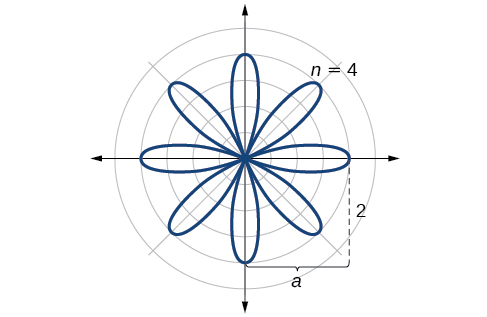Rose curve,   n   even

Sketch the graph of $\text{\hspace{0.17em}}r=4\mathrm{sin}\left(2\theta \right).$

The graph is a rose curve, $\text{\hspace{0.17em}}n\text{\hspace{0.17em}}$ even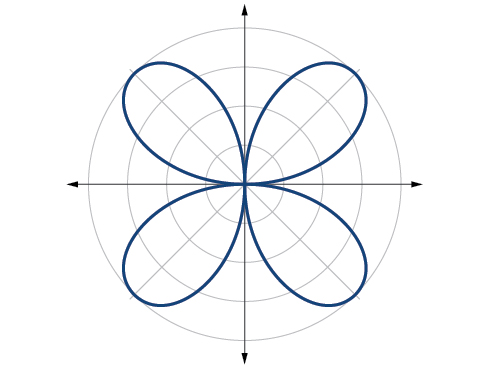## Sketching the graph of a rose curve ( n Odd)

Sketch the graph of $\text{\hspace{0.17em}}r=2\mathrm{sin}\left(5\theta \right).$

The graph of the equation shows symmetry with respect to the line $\text{\hspace{0.17em}}\theta =\frac{\pi }{2}.\text{\hspace{0.17em}}$ Next, find the zeros and maximum. We will want to make the substitution $\text{\hspace{0.17em}}u=5\theta .$

$\begin{array}{c}\text{\hspace{0.17em}}\text{\hspace{0.17em}}\text{\hspace{0.17em}}\text{\hspace{0.17em}}\text{\hspace{0.17em}}\text{\hspace{0.17em}}\text{\hspace{0.17em}}\text{\hspace{0.17em}}\text{\hspace{0.17em}}\text{\hspace{0.17em}}\text{\hspace{0.17em}}\text{\hspace{0.17em}}\text{\hspace{0.17em}}\text{\hspace{0.17em}}\text{\hspace{0.17em}}\text{\hspace{0.17em}}\text{\hspace{0.17em}}\text{\hspace{0.17em}}\text{\hspace{0.17em}}\text{\hspace{0.17em}}\text{\hspace{0.17em}}\text{\hspace{0.17em}}\text{\hspace{0.17em}}\text{\hspace{0.17em}}\text{\hspace{0.17em}}\text{\hspace{0.17em}}\text{\hspace{0.17em}}\text{\hspace{0.17em}}\text{\hspace{0.17em}}\text{\hspace{0.17em}}\text{\hspace{0.17em}}0=2\mathrm{sin}\left(5\theta \right)\\ \text{\hspace{0.17em}}\text{\hspace{0.17em}}\text{\hspace{0.17em}}\text{\hspace{0.17em}}\text{\hspace{0.17em}}\text{\hspace{0.17em}}\text{\hspace{0.17em}}\text{\hspace{0.17em}}\text{\hspace{0.17em}}\text{\hspace{0.17em}}\text{\hspace{0.17em}}\text{\hspace{0.17em}}\text{\hspace{0.17em}}\text{\hspace{0.17em}}\text{\hspace{0.17em}}\text{\hspace{0.17em}}\text{\hspace{0.17em}}\text{\hspace{0.17em}}\text{\hspace{0.17em}}\text{\hspace{0.17em}}\text{\hspace{0.17em}}\text{\hspace{0.17em}}0=\mathrm{sin}\text{\hspace{0.17em}}u\\ {\mathrm{sin}}^{-1}0=0\\ \text{\hspace{0.17em}}\text{\hspace{0.17em}}\text{\hspace{0.17em}}\text{\hspace{0.17em}}\text{\hspace{0.17em}}\text{\hspace{0.17em}}\text{\hspace{0.17em}}\text{\hspace{0.17em}}\text{\hspace{0.17em}}\text{\hspace{0.17em}}\text{\hspace{0.17em}}\text{\hspace{0.17em}}\text{\hspace{0.17em}}\text{\hspace{0.17em}}u=0\\ \text{\hspace{0.17em}}\text{\hspace{0.17em}}\text{\hspace{0.17em}}\text{\hspace{0.17em}}\text{\hspace{0.17em}}\text{\hspace{0.17em}}\text{\hspace{0.17em}}\text{\hspace{0.17em}}\text{\hspace{0.17em}}\text{\hspace{0.17em}}\text{\hspace{0.17em}}5\theta =0\\ \text{\hspace{0.17em}}\text{\hspace{0.17em}}\text{\hspace{0.17em}}\text{\hspace{0.17em}}\text{\hspace{0.17em}}\text{\hspace{0.17em}}\text{\hspace{0.17em}}\text{\hspace{0.17em}}\text{\hspace{0.17em}}\text{\hspace{0.17em}}\text{\hspace{0.17em}}\text{\hspace{0.17em}}\text{\hspace{0.17em}}\text{\hspace{0.17em}}\theta =0\end{array}$

The maximum value is calculated at the angle where $\text{\hspace{0.17em}}\mathrm{sin}\text{\hspace{0.17em}}\theta \text{\hspace{0.17em}}$ is a maximum. Therefore,

$\begin{array}{l}\begin{array}{l}\\ r=2\mathrm{sin}\left(5\cdot \frac{\pi }{2}\right)\end{array}\hfill \\ r=2\left(1\right)=2\hfill \end{array}$

Thus, the maximum value of the polar equation is 2. This is the length of each petal. As the curve for $\text{\hspace{0.17em}}n\text{\hspace{0.17em}}$ odd yields the same number of petals as $\text{\hspace{0.17em}}n,\text{\hspace{0.17em}}$ there will be five petals on the graph. See [link] .

Create a table of values similar to [link] .

 $\theta$ 0 $\frac{\pi }{6}$ $\frac{\pi }{3}$ $\frac{\pi }{2}$ $\frac{2\pi }{3}$ $\frac{5\pi }{6}$ $\pi$ $r$ 0 1 −1.73 2 −1.73 1 0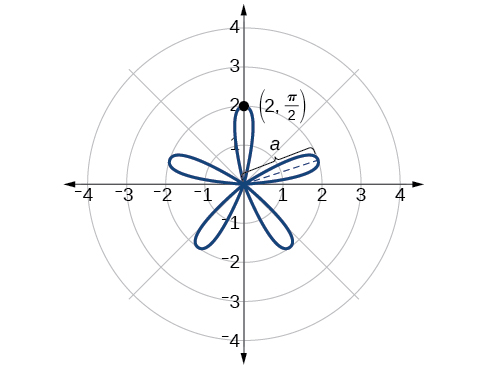Rose curve,   n   odd

Sketch the graph of $r=3\mathrm{cos}\left(3\theta \right).$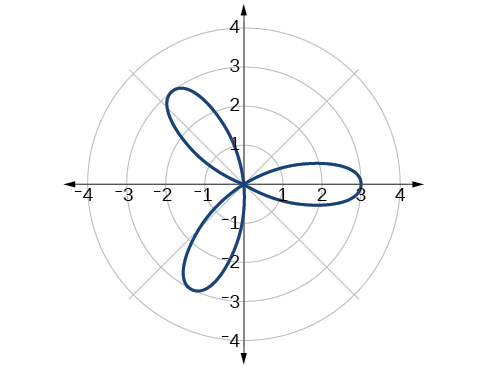Rose curve, $\text{\hspace{0.17em}}n\text{\hspace{0.17em}}$ odd

## Investigating the archimedes’ spiral

The final polar equation we will discuss is the Archimedes’ spiral, named for its discoverer, the Greek mathematician Archimedes (c. 287 BCE - c. 212 BCE), who is credited with numerous discoveries in the fields of geometry and mechanics.

## Archimedes’ spiral

The formula that generates the graph of the Archimedes’ spiral    is given by $\text{\hspace{0.17em}}r=\theta \text{\hspace{0.17em}}$ for $\text{\hspace{0.17em}}\theta \ge 0.\text{\hspace{0.17em}}$ As $\text{\hspace{0.17em}}\theta \text{\hspace{0.17em}}$ increases, $\text{\hspace{0.17em}}r\text{\hspace{0.17em}}$ increases at a constant rate in an ever-widening, never-ending, spiraling path. See [link] .

Given an Archimedes’ spiral over $\text{\hspace{0.17em}}\left[0,2\pi \right],$ sketch the graph.

1. Make a table of values for $\text{\hspace{0.17em}}r\text{\hspace{0.17em}}$ and $\text{\hspace{0.17em}}\theta \text{\hspace{0.17em}}$ over the given domain.
2. Plot the points and sketch the graph.

## Sketching the graph of an archimedes’ spiral

Sketch the graph of $\text{\hspace{0.17em}}r=\theta \text{\hspace{0.17em}}$ over $\text{\hspace{0.17em}}\left[0,2\pi \right].$

As $\text{\hspace{0.17em}}r\text{\hspace{0.17em}}$ is equal to $\text{\hspace{0.17em}}\theta ,\text{\hspace{0.17em}}$ the plot of the Archimedes’ spiral begins at the pole at the point (0, 0). While the graph hints of symmetry, there is no formal symmetry with regard to passing the symmetry tests. Further, there is no maximum value, unless the domain is restricted.

Create a table such as [link] .

 $\theta$ $\frac{\pi }{4}$ $\frac{\pi }{2}$ $\pi$ $\frac{3\pi }{2}$ $\frac{7\pi }{4}$ $2\pi$ $r$ 0.785 1.57 3.14 4.71 5.50 6.28

Notice that the r -values are just the decimal form of the angle measured in radians. We can see them on a graph in [link] .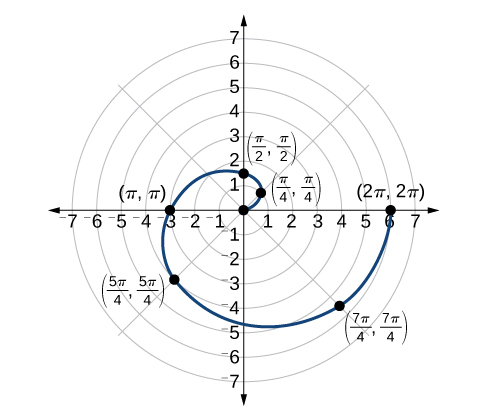Archimedes’ spiral

#### Questions & Answers

The sequence is {1,-1,1-1.....} has
amit Reply
circular region of radious
Kainat Reply
how can we solve this problem
Joel Reply
Sin(A+B) = sinBcosA+cosBsinA
Eseka Reply
Prove it
Eseka
Please prove it
Eseka
hi
Joel
June needs 45 gallons of punch. 2 different coolers. Bigger cooler is 5 times as large as smaller cooler. How many gallons in each cooler?
Arleathia Reply
find the sum of 28th term of the AP 3+10+17+---------
Prince Reply
I think you should say "28 terms" instead of "28th term"
Vedant
if sequence sn is a such that sn>0 for all n and lim sn=0than prove that lim (s1 s2............ sn) ke hole power n =n
SANDESH Reply
write down the polynomial function with root 1/3,2,-3 with solution
Gift Reply
if A and B are subspaces of V prove that (A+B)/B=A/(A-B)
Pream Reply
write down the value of each of the following in surd form a)cos(-65°) b)sin(-180°)c)tan(225°)d)tan(135°)
Oroke Reply
Prove that (sinA/1-cosA - 1-cosA/sinA) (cosA/1-sinA - 1-sinA/cosA) = 4
kiruba Reply
what is the answer to dividing negative index
Morosi Reply
In a triangle ABC prove that. (b+c)cosA+(c+a)cosB+(a+b)cisC=a+b+c.
Shivam Reply
give me the waec 2019 questions
Aaron Reply
the polar co-ordinate of the point (-1, -1)
Sumit Reply

### Read also:

#### Get the best Algebra and trigonometry course in your pocket!

Source:  OpenStax, Algebra and trigonometry. OpenStax CNX. Nov 14, 2016 Download for free at https://legacy.cnx.org/content/col11758/1.6
Google Play and the Google Play logo are trademarks of Google Inc.

Notification Switch

Would you like to follow the 'Algebra and trigonometry' conversation and receive update notifications?ByByByByBy Rhodes